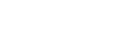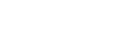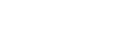# Can ChatGPT Solve Math Problems?

Looking for some help with numbers?Last Updated on

ChatGPT is a large language model developed by OpenAI that can generate human-like responses. It has been trained on massive text data and can answer various questions, but can ChatGPT solve math problems? The ease with which the AI model can solve advanced equations and calculations has left many people wondering if the chatbot can help out with their math homework. In this article, we will walk around the capabilities of ChatGPT in solving math problems.

## Mathematical Expression and Equations

Mathematical expressions and equations are a core mathematics component, and ChatGPT can process them. It can perform basic arithmetic operations such as addition, subtraction, multiplication, and division. It can solve more complex equations, such as quadratic equations and systems of linear equations.

#### Essential AI ToolsEXCLUSIVE DEAL 10,000 free bonus credits

Jasper AI

On-brand AI content wherever you create. 100,000+ customers creating real content with Jasper. One AI tool, all the best models.Copy.ai

Experience the full power of an AI content generator that delivers premium results in seconds. 8 million users enjoy writing blogs 10x faster, effortlessly creatingOnly \$0.00015 per word!

Winston AI detector

Winston AI: The most trusted AI detector. Winston AI is the industry leading AI content detection tool to help check AI content generated with ChatGPT,Only \$0.01 per 100 words

Originality AI detector

Originality.AI Is The Most Accurate AI Detection.Across a testing data set of 1200 data samples it achieved an accuracy of 96% while its closest competitorWordAI

10x Your Content Output With AI. Key features – No duplicate content, full control, in built AI content checker. Free trial available.

## Solving Simple & Complex Math Problems

ChatGPT can solve simple math problems such as addition, subtraction, multiplication, and division. For example, if you ask ChatGPT what 5+5 is, it will respond with the correct answer, 10. Similarly, asking what 20-5 is, it will respond with an accurate answer of 15.

In addition to this, ChatGPT can solve more complex math problems such as calculus, differential equations, geometry, and trigonometry. It can use deep learning algorithms to understand the problem and generate a solution. However, due to its nature as a natural language model, it may not always be able to solve the problem correctly, especially if it is too complex or requires a specific method or formula. Although it will be able to perform math to a certain level of accuracy, ChatGPT undoubtedly excels more at essays and essay writing.

## Using ChatGPT to Learn Math

ChatGPT can provide step-by-step solutions to math problems and explain the underlying concepts and formulas. This can help students and professionals understand the logic behind the solution and improve their math skills. Additionally, ChatGPT can generate many math practice problems for students to complete, and lead to their improvement. The use of new ChatGPT plug-ins, such as the custom instructions, can enable the chatbot to act more directly as a teacher. Using this plug-in can help to make the most of the potential that this platform has to offer students, but they are currently only available with a ChatGPT Plus subscription.

## Limitations of ChatGPT in Solving Math Problems

While ChatGPT is a powerful tool for solving math problems, it has limitations. One limitation is that it may not always be able to solve complex math equations that require a specific method or formula. Additionally, it may not always provide the most efficient solution to a problem. Moreover, it may not solve problems requiring real-world knowledge or context, as logical reasoning remains one of the limitations of ChatGPT.

On the flip side, the capabilities of ChatGPT will only ever expand and improve. As the successor to GPT-3, GPT-4 is an advanced multimodal model that outshines ChatGPT in many ways, including its math problem-solving abilities. As a large language model, math is not the forte of GPT-4. However, it still performs at high difficulty levels, and further updates to the GPT model will only see it perform better math and more advanced.

When you blend the pure arithmetic of a calculator with the expressiveness of a neural network, it turns out you get the worst of both worlds. A calculator that sometimes just makes things up.

Notice how, in the image above, ChatGPT fails to replace every instance of the number 5 with the number 6. There are two “5”s, but the AI chat bot only replaced the first of them.

In addition to this linguistic lack of attention to detail, the answer was objectively wrong anyway.

513 * 71.378 = 36616.914

This then divided by 9.3567 equals 3913.44320113

Not 3915.181, as calculated by ChatGPT.

What we see here is that ChatGPT is not only limited by decimal length in output, but internally truncated to keep server costs down. As a result, the errors from decimal shortening carry over, giving us an incorrect answer.

## Conclusion

ChatGPT is a powerful tool for solving math problems. It can solve simple and complex math problems in a matter of seconds, give explanations of concepts and formulas, and provide step-by-step solutions.

However, it has limitations and may not always be able to solve complex problems or provide the most efficient solution. Nonetheless, Chat GPT can be useful for learning and practicing math problems. Therefore, it is handy for solving various math problems and explaining their solutions in detail.

If you’re truly interested in using AI to help you directly with math, there are several options you can check out if you’re concerned the limitations of Chat GPT will be a significant issue. These additional resources include Wolfram Alpha, which is available as a Wolfram Plugin for ChatGPT Plus, and the Photomath app.